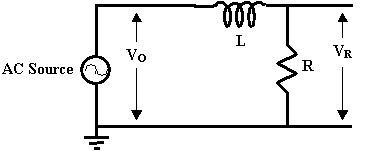# Physics 109 - RL Time Constants

In this experiment you will study another physical system that exhibits an exponential decrease as a function of time. The combination of a resistor R and inductor L in series exhibits an exponential transient response which you can observe by measuring the volage across the resistor in the circuit below.Instead of observing a decay in a single shot by opening a switch, you will repeatedly trigger the transient by using the AC function generator to generate SQUARE WAVES. Every time the voltage of the generator jumps up or down, you will see the transient response to the sudden change.

• Assemble the circuit. IMPORTANT NOTE: make sure that the ground connections of the oscilloscope and the function generator are the same.
• Set the function generator to 10 Hz square waves
• Initially, try a resistance of 100 Ohms
• You can trigger the oscilloscope externally by connecting the 'SYNC' output of the function generator to the 'EXT TRIG' connection on your oscilloscop. Set the trigger source to External.
• Make sure channel 1 being used for the measurements is DC coupled.
• When you get the scales adjusted to see the exponential decay, treat the voltage it decays to as being zero volts and do all voltage measurments relative to that level.
• tau is the time it takes for the voltage to drop from its initial voltage Vo down to a volatge of Vo/e
• Good precision measurments are obtained if you stretch the time scale to use a large fraction of the screen for your time measurements

Theoretically, the time constant, tau, in this circuit is given by the ratio L/R. The goal of the experiment is to check this theory and determine the value of the inductance L. You should initially use a range of resistance values between 10 and 2000 Ohms and take at least 10 measurements. Plot 1/tau versus R for your initial check of the relationship.

Marking Scheme

11 marks: includes high quality measurement of time constant versus resistance, plotting and fitting a model to the data, and conclusions